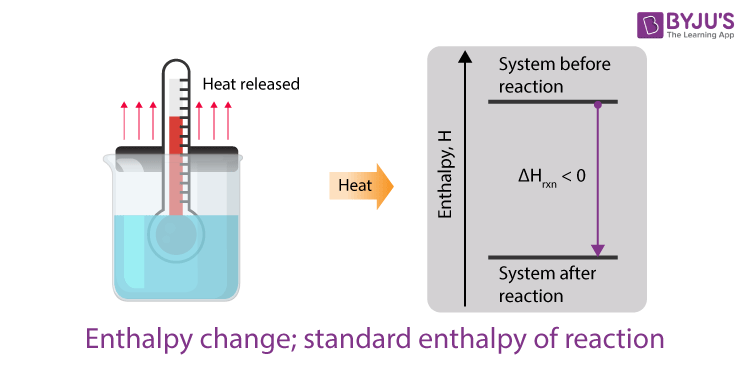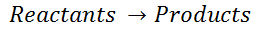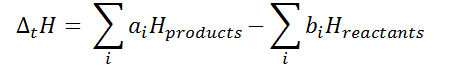# Enthalpy Change: Standard Enthalpy of Reaction

## IntroductionWhile dealing with a chemical reaction, the knowledge of enthalpy and standard enthalpy is very important. It helps in calculating the temperature and pressure required for any chemical reaction. It is also required for calculating the amount of heating and cooling necessary when the reaction is carried out commercially (at a large scale), for example, for the mass production of any component such as ammonia, calcium carbonate, oxygen etc. In this section, we will learn about the calculation of the change of enthalpy for a chemical reaction.

## Enthalpy change

Enthalpy change is the standard enthalpy of formation, which has been determined for a vast number of substances. In any general chemical reaction, the reactants undergo chemical changes and combine to give products. It can be represented by the following equation:For any such reaction, the change in enthalpy is represented as ΔrH and is termed the reaction enthalpy.  The reaction enthalpy is calculated by subtracting the sum of enthalpies of all the reactants from that of the products.

Mathematically,

ΔtH = Sum of enthalpies of the product – sum of the enthalpies of the reactants.Here, the constants ai and bi denotes the stoichiometric coefficients of the products and the reactants respectively for the balanced chemical reaction under consideration.

## Standard enthalpy of reaction

We already know the enthalpy of any reaction depends on the physical conditions of the surroundings such as the temperature, pressure, etc. In order to specify the standard enthalpy of any reaction, it is calculated when all the components participating in the reaction i.e., the reactants and the products are in their standard form. Therefore the standard enthalpy of reaction is the enthalpy change that occurs in a system when a matter is transformed by a chemical reaction under standard conditions.

As per convention, the standard state for any substance at a specified temperature is its pure form at a pressure of 1 bar. For an example, liquid ethanol at 298 K and 1 bar of pressure are said to be in its standard state in its pure form. It is important to note that the data for the standard state for a substance is taken at 298K. The standard enthalpy of a reaction is denoted as ΔrHs. At constant pressure, the heat of the reaction is exactly equal to the enthalpy change, of the reacting system.

## Frequently Asked Questions – FAQs

Q1

### What do you mean by enthalpy of formation?

Formation enthalpy is the normal reaction enthalpy for the formation of the compound from its elements (atoms or molecules) at the chosen temperature (298.15K) and at 1 bar pressure in their most stable reference states.
Q2

### What is the difference between the standard enthalpy of reaction and standard enthalpy of formation?

The normal formation of enthalpy is the transition in enthalpy that accompanies from its elements the formation of one mole of the compound. The normal reaction enthalpy happens in a system where a chemical reaction converts one mole of matter.
Q3

### What is enthalpy in simple terms?

Enthalpy is a term used in science and engineering where it is required to quantify heat and function. At constant strain, as a material varies, enthalpy informs how much heat and effort has been applied or extracted from the substance. Enthalpy is energy-like, but not the same.
Q4

### Write the expression for the change in enthalpy.

The reaction enthalpy is calculated by subtracting the sum of enthalpies of all the reactants from that of the products.
Mathematically,
ΔtH = Sum of enthalpies of the product – sum of the enthalpies of the reactants.
Take up a quiz on Enthalpy Change: Standard Enthalpy of Reaction